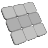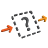Back to Adaptive Vision Studio website

You are here: Start » Filter Reference » Matrix

Matrix

Select a filter from the list below.

IconNameDescriptionAccessMatrixReturn matrix dimensions and its elements row-by-row as a real array.AddMatricesAdd two matrices of identical dimensionsCombineMatricesCombine two matrices horizontallyConcatenateMatricesCombine two matrices horizontallyCreateIdentityMatrixCreate a square matrix with ones on the main diagonal, and zeros elsewhere.CreateUniformMatrixCreate a matrix with the specified dimensions and filled with an uniform element value.GetMatrixColumnGets the values from a single column of a matrix.GetMatrixElementGets the value of a single element of a matrix.GetMatrixRowGets the values from a single row of a matrix.InvertMatrixFind the inverse of a square matrix.MakeMatrixCreate a matrix of specified dimensions with initial elements given row-by-row.MatrixToRealArrayArrayConverts a matrix to an array of its rows.MultiplyMatricesCombine two matrices horizontallyMultiplyMatrixByRealMultiply all elements of a matrix by a value.SetMatrixColumnSets elements of a matrix column.SetMatrixElementSets a single element of a matrix.SetMatrixRowSets elements of a matrix row.SkipEmptyMatrixIf the input matrix dimensions are non-zero, then the matrix is copied to the output; otherwise Nil value is returned.StackMatricesCombine two matrices verticallySubtractMatricesSubtract two matrices of identical dimensionsTransposeMatrixFind the transpose of a matrix.